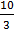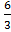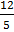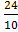### Sample Problem

Find the simplest form of the following ratios.

(a):=

(b):#### Solution

Always express ratios in term of whole numbers in their lowest terms. Thus,

First, multiply each part of the ratio by a same factor to make them whole numbers.

Then, if the ratio is not at its simplest form, divide each whole number of the given ratios by common factors until they cannot be divided by any factors.

(a):= (× 15) : (× 15) = 30:36

30:36 = (30 ÷ 6) : (36 ÷ 6) = 5:6

(b):= (× 30) : (× 30) = 72:100

72:100 = (72 ÷ 4) : (100 ÷ 4) = 18:25